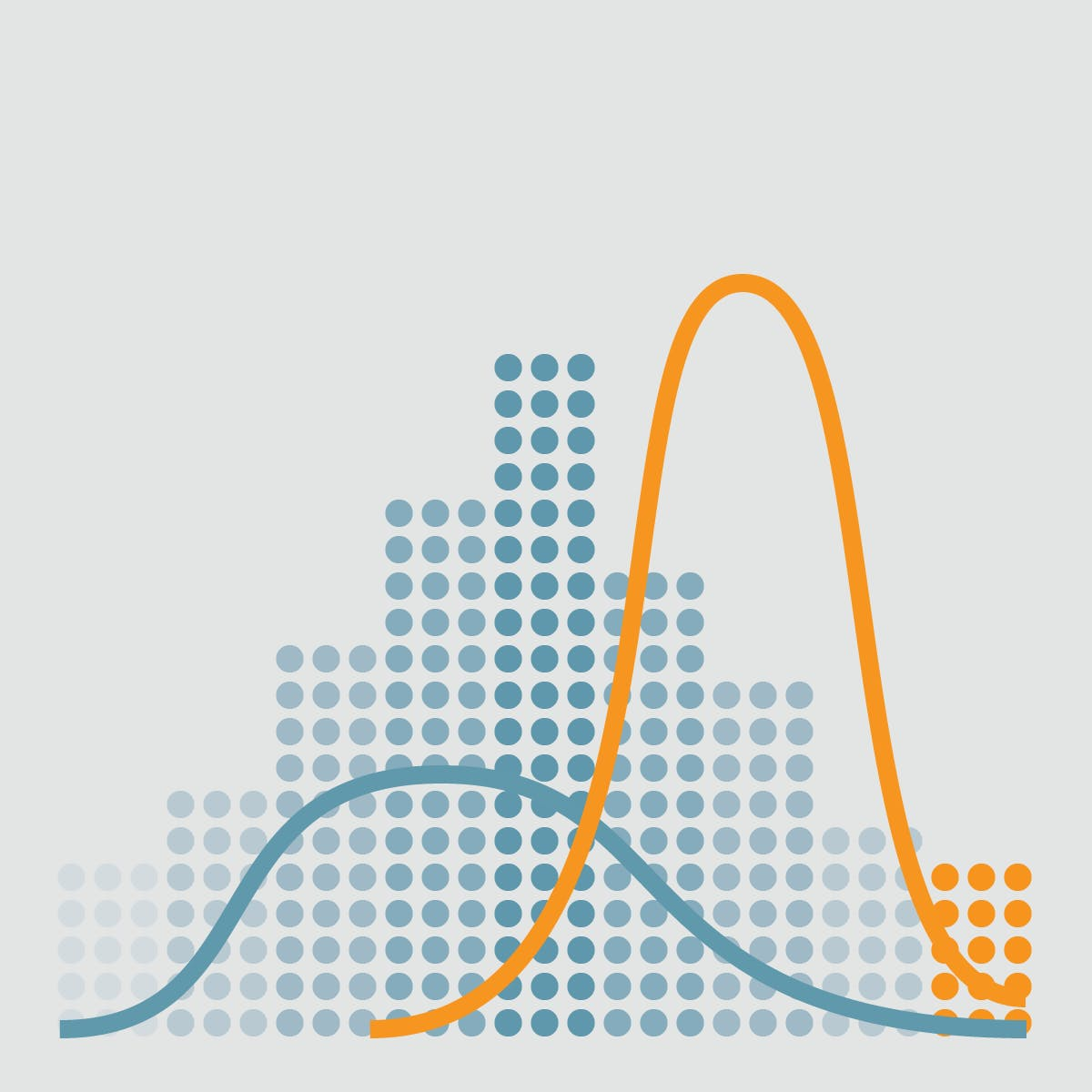# 2.4 - Estimates of Location

Last updated: March 14th, 2019# Estimates of Location¶

Variables with measured or count data might have thousands of distinct values. A basic step in exploring your data is getting a "typical value" for each feature (variable): an estimate of where most of the data is located (i.e., its central tendency).## Hands on!¶

In [ ]:
import numpy as np
import pandas as pd
import matplotlib.pyplot as plt
import seaborn as sns

%matplotlib inline


We'll use the following Google Play Store Apps dataset in this lesson:

In [ ]:
df = pd.read_csv('data/googleplaystore.csv')

df.head()### Measuring Central Tendency¶

Mathematically, central tendency means measuring the center or distribution of location of values of a data set. It gives an idea of the average value of the data in the data set and also an indication of how widely the values are spread in the data set.

That in turn helps in evaluating the chances of a new input fitting into the existing data set and hence probability of success.

#### Frequency distribution of a variable¶

The first thing we're going to do is plot a line of every sample value of the Rating column:

In [ ]:
df['Rating'].plot(color='#3498db', figsize=(12,6))


#### Histogram¶

It's a mess, so we're going to make an accurate representation of the distribution of the sample values by getting the frequency of each value.

In [ ]:
freq = df['Rating'].value_counts().sort_index()

freq_frame = freq.to_frame()

freq_frame.plot.bar(color='#3498db', figsize=(12,6))


This plot of the frequency (count) of the values is known as a Histogram:

In [ ]:
df['Rating'].plot.hist(bins=20, color='#3498db', figsize=(12,6))### Density estimates¶

Related to the histogram is a Density plot, which shows the distribution of data values as a continuous line.

This density plot can be thought of as a smoothed version of a histogram, although it is typically computed directly from the data through a kernel density estimate.

We'll use Seaborn library to make our plots:

In [ ]:
plt.figure(figsize=(12,6))

sns.distplot(df['Rating'].dropna())### Mean¶

The sum of all values divided by the number of values. Also known as average. This is the most basic estimate of location.

The formula to compute the mean for a set of $n$ values $x_1, x_2, ..., x_n$ is:

$$Mean = \mu = \overline{x} = \frac{\sum\limits_{i}^n x_i }{n}$$

Let's calculate the mean of the Rating of Google Play Store Apps.

In [ ]:
mean_rating = df['Rating'].mean()

mean_rating

In [ ]:
plt.figure(figsize=(12,6))

sns.distplot(df['Rating'].dropna())

# Mean line
plt.axvline(mean_rating, color='#e74c3c', linestyle='dashed', linewidth=2)### Median¶

The value such that one-half of the data lies above and below. If there is an even number of data values, the middle value is one that is not actually in the data set, but rather the average of the two values that divide the sorted data into upper and lower halves.

Compared to the mean, which uses all observations, the median depends only on the values in the center of the sorted data.

The median will be a robust estimator of location since it is not influenced by outliers that could sked the results.

In [ ]:
median_rating = df['Rating'].median()

median_rating

In [ ]:
plt.figure(figsize=(12,6))

sns.distplot(df['Rating'].dropna())

# Mean line
plt.axvline(mean_rating, color='#e74c3c', linestyle='dashed', linewidth=2)

# Median line
plt.axvline(median_rating, color='#e67e22', linestyle='dashed', linewidth=2)### Mode¶

The most commonly occurring category or value in a data set.

In [ ]:
mode_rating = df['Rating'].mode()

mode_rating

In [ ]:
plt.figure(figsize=(12,6))

sns.distplot(df['Rating'].dropna())

# Mean line
plt.axvline(mean_rating, color='#e74c3c', linestyle='dashed', linewidth=2)

# Median line
plt.axvline(median_rating, color='#e67e22', linestyle='dashed', linewidth=2)

# Mode line
plt.axvline(mode_rating, color='#f1c40f', linestyle='dashed', linewidth=2)